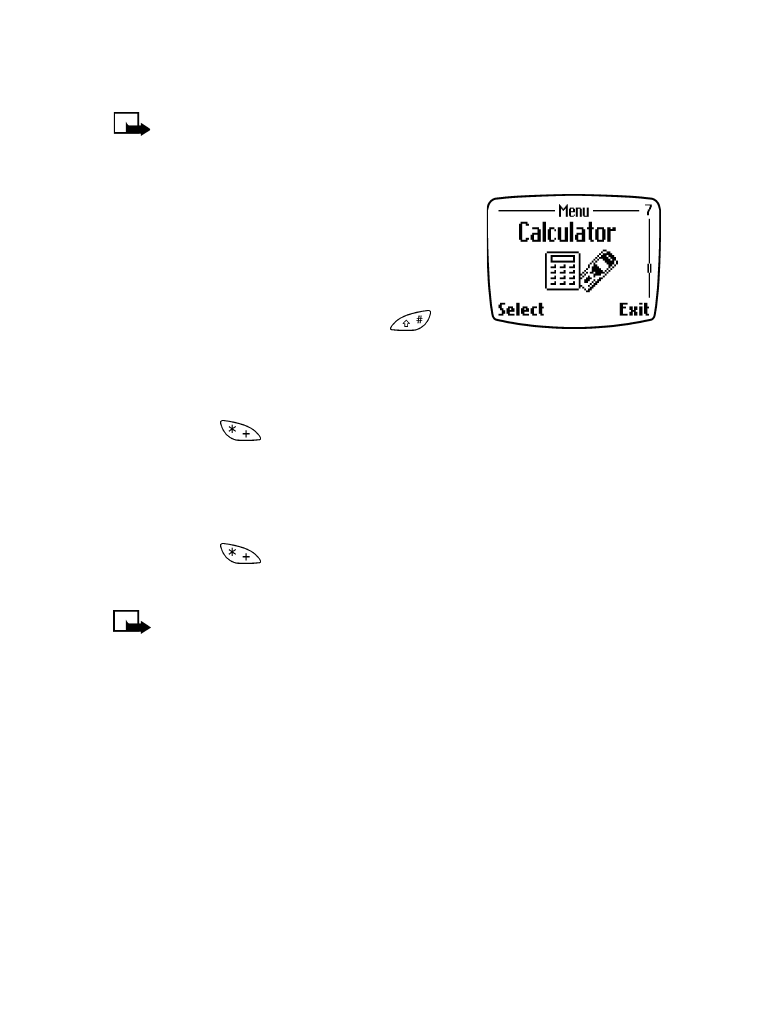## Nokia 8270 - • Calculator2

Enter the first number in the equation.

(To enter a decimal point, press

.

To clear one digit, press Clear. To clear
all digits, press and hold Clear.)

3

Enter the symbol: (+ add), (- subtract), (* multiply), or (/ divide):

Press

as many times as necessary, OR...

Press Options. Scroll to Add, Subtract, Multiply, or Divide. Press OK.

4

Enter the second number in the equation.

5

Calculate the result:

Press ,

OR...

Press Options. Scroll to Equals. Press OK.

Note: This calculator has a limited accuracy and rounding errors
may occur, especially in long divisions.[ 95 ]

Games